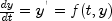JMSLTM Numerical Library 7.2.0
com.imsl.math

• All Superinterfaces:
Enclosing class:

```public static interface OdeAdamsGear.Jacobian
Public interface for the user supplied function to evaluate the Jacobian matrix.
• ### Method Summary

Methods
Modifier and Type Method and Description
`double[][]` ```jacobian(double t, double[] y, double[] yprime)```
Used to compute the Jacobian of the function at `t`.
• ### Methods inherited from interface com.imsl.math.OdeAdamsGear.Function

`f`
• ### Method Detail

• #### jacobian

```double[][] jacobian(double t,
double[] y,
double[] yprime)```
Used to compute the Jacobian of the function at `t`.
Parameters:
`t` - a `double`, the point at which the function is to be evaluated. (Input)
`y` - a `double` array which contains the dependent variable values. (Input)
`yprime` - a `double` array which contains the value of the function. (Input)
Returns:
a `double` `y.length` by `y.length` matrix containing the value of the Jacobian of the function at `t`. (Output)
JMSLTM Numerical Library 7.2.0